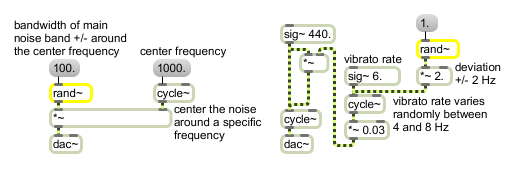# rand~

Band-limited random signal

## Description

rand~ generates a signal consisting of random values between -1 and 1 generated at a frequency specified by its input. It interpolates linearly between these values.

## Arguments

Name Type Opt Description
initial-frequency (Hz) float or int opt Sets the initial frequency. The default value is 0. If a signal is connected to the inlet, the argument is ignored.

## Messages

 int input [int] Same as signal. If there is a signal connected to the inlet, a float or int is ignored. float input [float] Same as signal. If there is a signal connected to the inlet, a float or int is ignored. signal The frequency at which a new random number between -1 and 1 is generated. rand~ interpolates linearly between random values chosen at the specified rate.

## Output

signal: A signal consisting of line segments between random values in the range -1 to 1. The random values occur at the frequency specified by the input.

## ExamplesUse rand~ to create roughly band-limited noise or as a control signal to create random variation